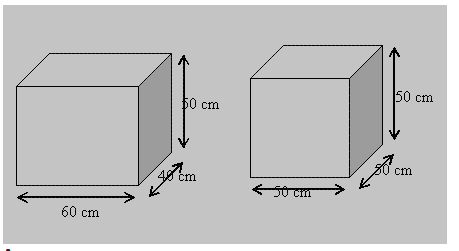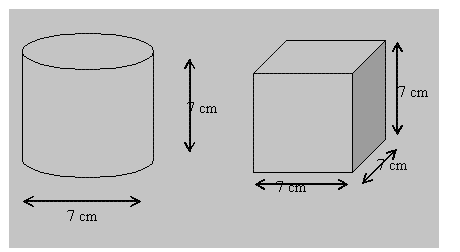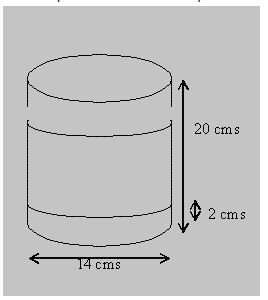# Mensuration class 8 solutions 11.3

In this page we have mensuration class 8 solutions 11.3 for Chapter 11 . This exercise has questions about Solid shapes like cube, cubiod and cylinder,How to find the surface area of them.Hope you like them and do not forget to like , social share and comment at the end of the page.

## Mensuration class 8 solutions 11.3

Question 1
There are two cuboidal boxes as shown in the adjoining figure. Which box requires the lesser amount of material to make?Volume of the Cuboid   is given by
= Lx B x H
= 60 x 40 x 50 = 12000 cm3
Volume of Cube = Side3
=503 = 125000 cm3
As the volume of the cube is greater than that of the cuboid so it will require more material, and the cuboid will require less material

Question 2
A suitcase with measures 80 cm × 48 cm × 24 cm is to be covered with a tarpaulin cloth. How many metres of tarpaulin of width 96 cm is required to cover 100 such suitcases?
Surface Area of Cuboid is given by
= 2(LB + LH + BH)
= 2 (80 x 48 + 80 x 24 + 48 x 24)
= 2(3840 + 1920 + 1152)
= 2 x 6912 = 13824
Hence, Surface Area of 100 suitcases = 1382400 cm2
Required tarpaulin will have same area
So required length of tarpaulin= Area /width
=1382400/96= 14400 cm=144 m

Question 3
Find the side of a cube whose surface area is 600 cm2.
Let a be the side
Surface Area = 6 x side2
600 = 6 x a2
a2 = 100
a = 10 cm

Question 4
Rukhsar painted the outside of the cabinet of measure 1 m × 2 m × 1.5 m. How much surface area did she cover if she painted all except the bottom of the cabinet?
Surface Area of Cabinet = 2(LB + LH + BH)
= 2(1 x 2 + 1 x 1.5 + 1.5 x 2)
= 2(2 + 1.5 + 3) = 2 x 6.5 = 13
Area of Bottom Surface = 1 x 2 = 2 m2
Hence, Area Covered by painting = 13-2 = 11 m2

Question 5
Daniel is painting the walls and ceiling of a cuboidal hall with length, breadth and height of 15 m, 10 m and 7 m respectively. From each can of paint 100 m² of area is painted. How many cans of paint will she need to paint the room?
Area of All walls, ceilings and floor = 2(LB + LH + BH)
= 2(15 x 10 + 15 x 7 + 10 x 7)
= 2(150 + 105 + 70)
= 2 x 325 = 650
Area of Floor = 15 x 10 = 150
Hence, Painted Area =650-150=500
As, one can of paint is enough for 100 sq m
So, 500/100 = 5 cans are needed to paint the hall.

Question 6
Describe how the two figures given below are alike and how they are different. Which box has larger lateral surface area?The similarity is that their dimensions are equal
The difference is the figure on the left is cylindrical and that on the right is cuboidal.
Lateral Surface area of cylinder= 2πrh=  154 cm2
Surface Area of Cube= 4×side=  196 cm2
It is clear that the cylinder is having a larger surface area.

Question 7
A closed cylindrical tank of radius 7 m and height 3 m is made from a sheet of metal. How much sheet of metal is required?
Surface Area of cylinder= 2πrh + 2πr2
=440 m2

Question 8
The lateral surface area of a hollow cylinder is 4224 cm2. It is cut along its height and formed a rectangular sheet of width 33 cm. Find the perimeter of rectangular sheet?
Area of Rectangular Sheet = Surface Area of the Cylinder
Area of Rectangle = Lx B
Or, Lx B = 4224
Lx 33 = 4224
L=128 cm

Question 9
A road roller takes 750 complete revolutions to move once over to level a road. Find the area of the road if the diameter of a road roller is 84 cm and length is 1 m.
In one revolution the wheel of the roller will cover an area equal to its surface area.
Curved Surface Area of cylinder=2 πrh
=26400 cm2= 2.64 m2
Question 10
A company packages its milk powder in cylindrical container whose base has a diameter of 14 cm and height 20 cm. Company places a label around the surface of the container (as shown in the figure). If the label is placed 2 cm from top and bottom, what is the area of the label.Answer: The cylindrical area covered by the label will have
Height = 20-4 = 16 cm
Curved Surface area of cylinder= 2 πrh
=704 cm2

## Summary

1. Mensuration class 8 solutions 11.3 has been prepared by Expert with utmost care. If you find any mistake.Please do provide feedback on mail. You can download the solutions as PDF in the below Link also
2. This chapter 11 has total 4 Exercise 11.1 ,11.2 ,11.3 and 11.4. This is the third exercise in the chapter.You can explore previous exercise of this chapter by clicking the link below